# Leveraging Generated Code from MATLAB in a C++ Application

Today I am happy to welcome guest blogger Erin McGarrity. The main reason why I am excited to welcome Erin as guest blogger is that he will be talking about generating C/C++ code from MATLAB code and Simulink models using MATLAB Coder, Simulink Coder and Embedded Coder. While I have historically focused more on simulation-related topics on this blog, I realize that code generation is an important topic for many readers of this blog. We would like to hear in the comments below what you think of today's topic, and what other code generation related topics you would like to see covered on this blog.

## Introduction

Let's say we have a fancy numerical routine that we've been working on in MATLAB that we want to utilize in an external C++ application. In this post, we're going to show how we can generate a dynamic library from MATLAB code which we can call from a C++ program. This will allow us to harness some of the power of MATLAB in an external program.

## The MATLAB Code

To demonstrate the workflow, let's create a routine that performs a line fit to some data. This function will have 2 inputs, x and y, which are both vectors of data to fit. These vectors should have the same length N. The outputs for this function are m and b, the slope and intercept of the line that best fits the data. We'll perform our fit by minimizing the squares of the vertical distances of the points to the line in the point-slope form which is given by this equation :
$\varphi \left(\mathit{m},\mathit{b}\right)=\text{\hspace{0.17em}}\sum _{\mathit{i}=1}^{\mathit{N}}{\left(\mathit{y}\left(\mathit{i}\right)-\left(\mathit{m}\cdot \mathit{x}\left(\mathit{i}\right)+\mathit{b}\right)\right)}^{2}$
The minimum of $\varphi \left(\mathit{m},\mathit{b}\right)\text{\hspace{0.17em}}$can be found using the usual technique of taking the derivative of it with respect to m and b and setting these to zero. Doing so gives us the following system of 2 equations:
$\frac{\partial }{\partial \mathit{m}}\varphi \left(\mathit{m},\mathit{b}\right)=0=\sum _{\mathit{i}=1}^{\mathit{N}}-2\left(\mathit{y}\left(\mathit{i}\right)-\left(\mathit{m}\cdot \mathit{x}\left(\mathit{i}\right)+\mathit{b}\right)\right)\cdot \mathit{x}\left(\mathit{i}\right)$
and
$\frac{\partial }{\partial \mathit{b}}\varphi \text{\hspace{0.17em}}\left(\mathit{m},\mathit{b}\right)=0=\sum _{\mathit{i}=1}^{\mathit{N}}-2\left(\mathit{y}\left(\mathit{i}\right)-\left(\mathit{m}\cdot \mathit{x}\left(\mathit{i}\right)+\mathit{b}\right)\right)\cdot 1$
Here we have used the fact that the derivative operator and sum commute. After dividing both sides of both equations by -2, we can rearrange this system into a matrix equation
$\left[\begin{array}{cc}\sum _{\mathit{i}=1}^{\mathit{N}}{\mathit{x}\left(\mathit{i}\right)}^{2}& \sum _{\mathit{i}=1}^{\mathit{N}}\mathit{x}\left(\mathit{i}\right)\\ \sum _{\mathit{i}=1}^{\mathit{N}}\mathit{x}\left(\mathit{i}\right)& \mathit{N}\end{array}\right]\cdot \left[\begin{array}{c}\mathit{m}\\ \mathit{b}\end{array}\right]=\left[\begin{array}{c}\sum _{\mathit{i}=1}^{\mathit{N}}\mathit{x}\left(\mathit{i}\right)\cdot \mathit{y}\left(\mathit{i}\right)\\ \sum _{\mathit{i}=1}^{\mathit{N}}\mathit{y}\left(\mathit{i}\right)\end{array}\right].$
This system can be written in compact notation as
$\mathit{H}\cdot \mathit{p}=\mathit{f}.$
To solve for $p=[m,b]^T$ , we can multiply both sides by the inverse of H
${\mathit{H}}^{-1}\cdot \mathit{H}\cdot \mathit{p}={\mathit{H}}^{-1}\cdot \mathit{f},$
or simply
$\mathit{p}={\mathit{H}}^{-1}\cdot \mathit{f}.$
The MATLAB code to solve for m and b looks like this:
function [m, b] = lineFitter(x,y)

% Make sure x and y have the same number of elements
assert(numel(x)==numel(y));
% Form the left hand matrix
H = [x(:)'*x(:) sum(x(:),1);
sum(x(:),1) numel(x)];

% Form the residual
f = [x(:)'*y(:)
sum(y(:),1)];

% Solve the system
p = H\f;
% Assign the slopes
m = p(1);
b = p(2);
end

## Testing the Fitting Routine In MATLAB

To check our code, let's load some data, fit the line and plot everything.
x = d(:,1);
y = d(:,2);
% Fit the data
[m,b] = lineFitter(x,y)
m = 4.0493
b = -0.0247
% Create the line from the fitted parameters
xgrid = 0:0.1:1;
ygrid = m*xgrid + b;
% Plot everything
figure;clf
scatter(x,y);
hold all
plot(xgrid, ygrid, "LineWidth", 2);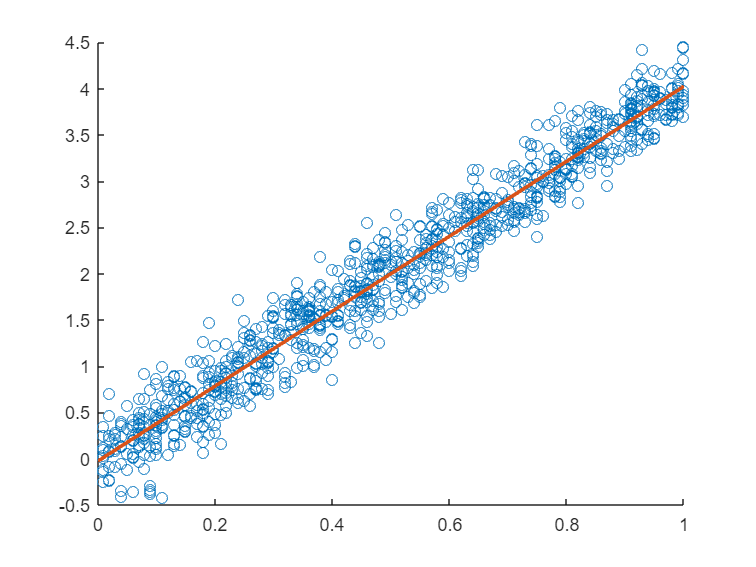## Generate Code for the Fitting Routine

Now we can generate code and everything we'll need to create a dynamic library from our lineFitter routine. We will use coder.config and coder.typeof
% Set up coder object for a dynamic library
cfg = coder.config('dll');
cfg.Verbosity='Verbose';
cfg.GenCodeOnly = true;

% Create sample variables for the inputs. We want to make these inputs
% dynamically sized because we may have arbitrarily sized data files.
xarg = coder.typeof(1.0, [10000, 1], [1, 0]);
yarg = coder.typeof(1.0, [10000, 1], [1, 0]);

% Generate the code and a report
codegen -config cfg lineFitter.m -args {xarg,yarg} -nargout 2 -report
### Compiling function(s) lineFitter ... ------------------------------------------------------------------------ Compilation suppressed: generating code only. ------------------------------------------------------------------------ ### Generating compilation report ... Code generation successful: View report
The code generation report in MATLAB R2022b has a neat feature called "Trace Code" that shows what MATLAB code corresponds to what C code: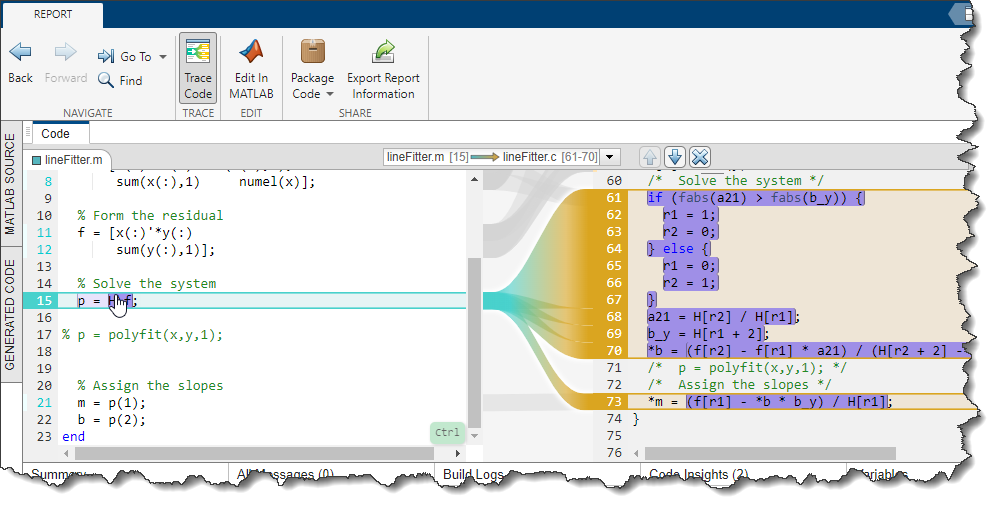The relevant code that we'll need later is the interface to lineFitter. The key code is in lineFitter.h:
/* Function Declarations */
extern void lineFitter(const emxArray_real_T *x, const emxArray_real_T *y,
double *m, double *b);
The inputs are constant array pointers and the outputs are pointers to doubles. This will guide our client-side implementation as we will show below.

## Packaging the Code

Now that we have our code, we can use codebuild and packNgo to generate and package build artifacts that we can take over to our client application. In this case we'll use the CMake and SHARED_LIBRARY options to codebuild so that we'll be able to use the CMake program to build everything on our client side. CMake is a tool that allows us to perform platform independent compilation of our code. In short, we can provide CMake with a list of things to build and it will perform all the checks and compilation for us.
codebuild('./codegen/dll/lineFitter', 'BuildMethod', 'CMake', 'BuildVariant', 'SHARED_LIBRARY');
packNGo('./codegen/dll/lineFitter', 'PackType', 'hierarchical', 'fileName', 'lineFitter');
This generates an archive file lineFitter.zip, which we can copy to our client codebase.

## Create the Dynamic Library

Now that we have our code, we're ready to build it and create a routine to link to it on the client machine. After that we can add the code we need to call our line fitting routine in the client code. Let's say we put our newly generated lineFitter.zip here and unzip everything. Unzipping lineFitter.zip will produce two files, mlrFiles.zip and sDirFiles.zip. Unzipping these will create two directories, R2022b and codegen.
C:\work\lineFitExample> dir /w
[codegen] lineFitter.zip mlrFiles.zip [R2022b] sDirFiles.zip
These contain the required MATLAB headers and our generated code, respectively. To build these, we'll create a directory called build_lib, then call cmake with the following commands  (their results are shown as well):
C:\work\lineFitExample> mkdir build_lib

C:\work\LineFitExample> cmake -G "NMake Makefiles" -B ./build_lib -DCMAKE_BUILD_TYPE=Release -DMATLAB_ROOT=./R2022b -S./codegen/dll/lineFitter
-- The C compiler identification is MSVC 19.29.30137.0
<snip>
-- Build files have been written to: C:/work/LineFitExample/build_lib

C:\work\LineFitExample> cmake --build build_lib
[8/8] Linking CXX shared library codegen\dll\lineFitter\lineFitter_win64.dll
\#\#\# Created library: C:/work/LineFitExample/build_lib/codegen/dll/lineFitter/lineFitter_win64.dll

## Using the Dynamic Library

Now that we have generated our dynamic library lineFitter_win64.dll, it's time to create the application code that will use the lineFitter routine. Our client application reads in the csv file and places the columns into two lists: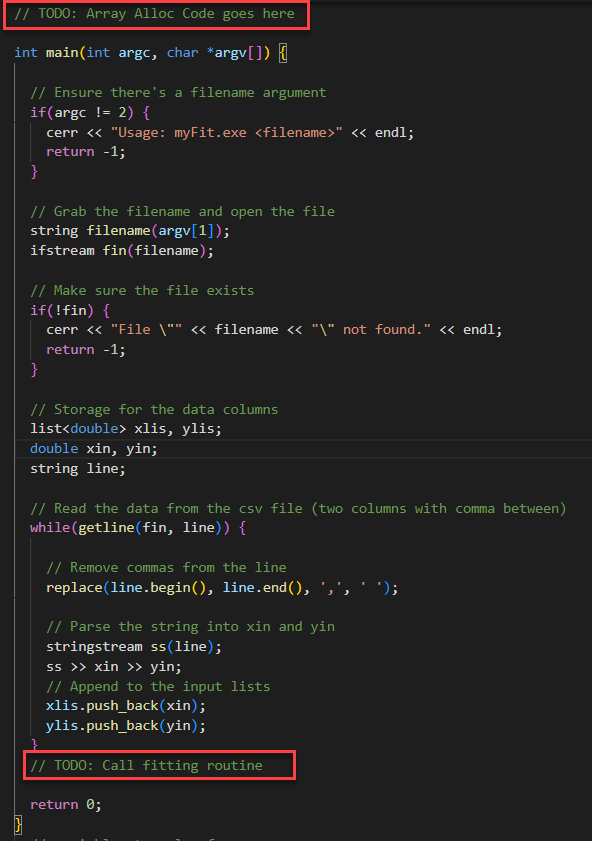To make this code interface with our newly created dynamic library we need to allocate our arrays. These arrays are of type emx_Array_real_T as shown above. We'll write a function to do the allocation, but first we'll include two header files that we'll need:
#include "lineFitter.h"
#include "lineFitter_emxAPI.h"
Now we're ready to write our array allocation function, which we'll place above the main routine. This function takes a list of doubles and copies it into an emxArray_real_T that has been allocated to the proper size. An example of this code can be found in the directory .\codegen\dll\lineFitter\examples\main.c.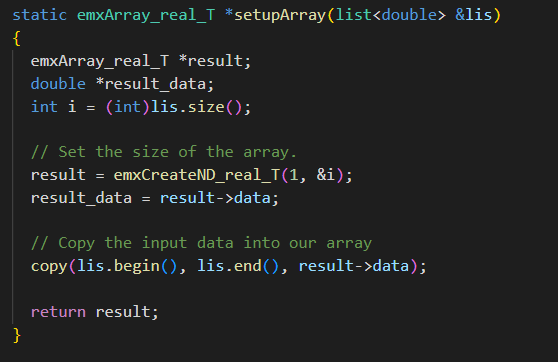Finally, we'll add the calls to allocate our vectors and solve the system below the reading code in the main routine but before the final return statement: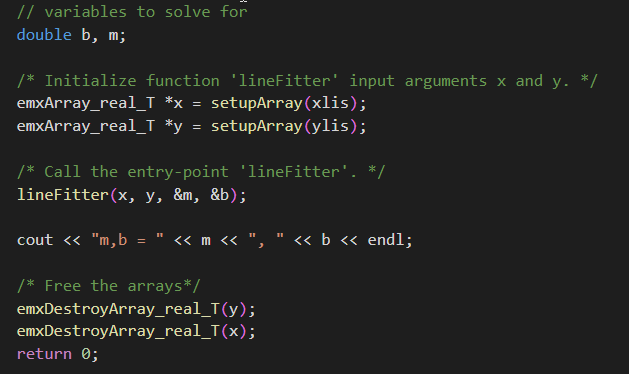Our CMakeLists.txt file for our client looks like this: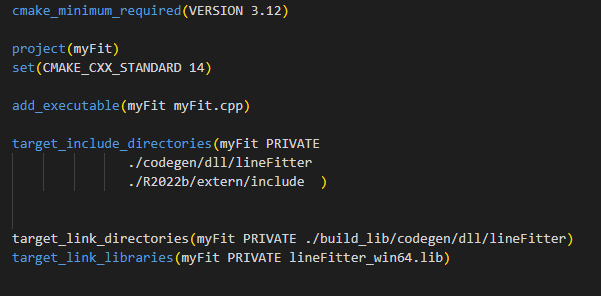Now we're ready to compile our client application. You can download the resulting files here: CMakeLists.txt and myFit.cpp. You'll also need the data file, which is here in myData.csv.
The procedure is similar to compiling the library above, which is part of the appeal of CMake. The commands are:
C:\work\LineFitExample> mkdir build

C:\work\LineFitExample> cmake -G "NMake Makefiles" -B build -S . -DCMAKE_BUILD_TYPE=Release
-- The C compiler identification is MSVC 19.29.30137.0
<snip>
-- Build files have been written to: C:/work/LineFitExample/build

C:\work\LineFitExample> cmake --build build
Now we have our executable, myFit.exe in the build directory. The final steps to test our code are:
C:\work\LineFitExample> set PATH=C:\work\LineFitExample\build_lib\codegen\dll\lineFitter\;%PATH%

C:\work\LineFitExample> .\build\myFit.exe myData.csv
m,b = 4.04926, -0.0246821
As we can see, the slope and intercept are the same as what we got in MATLAB.

## Notes

 This method is used for illustration purposes only. In production, you should use polyfit to solve this problem.
 In this example, we use Visual Studio tools to compile the code. To set this up for the command line, I ran the script:
C:\Program Files (x86)\Microsoft Visual Studio\2019\Professional\VC\Auxiliary\Build\vcvars64.bat